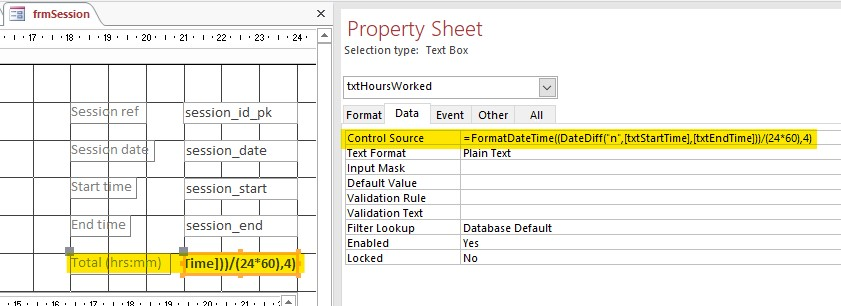## Saturday, 2 March 2019

### Converting Minutes to Hours:Minutes in MS Access

There may be occasions when we need to convert the difference between a start time and an end time, and display the result in hours and minutes.  For example, suppose we set up an Access table field to record the time a person starts work (the session_start field) and another to record when s/he finishes (the session_end) field.  Rather than set up a third field to store the total time worked, we can instead calculate this value automatically using a calculated control in an Access form, or a calculated field in an Access query.

 Above: the bottom text box (txtHoursWorked) is a calculated control.  The CONTROL SOURCE is an expression based on the values contained in the top two text boxes (bound to the session_start and session_end fields in the undelying table).
However, to do this we must use two expressions to calculate the time difference, and then display the time difference in the hrs:mm format.  So if we enter 20:40 as the session_start, and 22:06 for session_end, we need an expression to calculate the total minutes worked (which in this case is 86 minutes), and then convert the total minutes to hours/minutes format (which would be 01:26).  To achieve this we use the DateDiff and FormatDateTime functions.  Lets take a look at each function individually before combing them to produce the required result.

The syntax for the DateDiff function is as follows:

DateDiff("interval", time_1, time_2)

The function works by calculating the time difference between the time_1 and time_2 parameters, and returns the result in accordance with the interval parameter.  In our case, we want need the result returned as minutes, so enter "n" as interval.   Time_1 and Time_2 correspond to the start time and end time.  To get these values for the expression, we can we put a reference to the values contained in the text boxes for "Start time" and "End time".  In our example these text boxes are called txtStartTime and txtEndTime.  This what our DateDiff function looks once we add the parameter values:

DateDiff("n", txtStartTime, txtEndTime)

The value returned in our example is 86 minutes.

The second function that we are going to use is FormatDateTime which converts the value returned by the DateDiff function into hours and minutes.  The syntax we need to use in the FormatDateTime is as follows:

FormatDateTime( (minutes/(24*60) , named_format)

This first parameter is a division of the number of minutes (returned in the DiffFunction) by the total number of minutes in a day -  which in our example is 86/(24*60) - and returns the result in accordance with the value contained in the named_format parameter.  The latter is a numeric value indicating the required time format - which in our example is vbShortTime ie hours:minutes.  The numeric value we enter for the vbShortTime format is 4.  This is what our FormatDateTime function looks like once we add the parameter values:

FormatDateTime((86/(24*60) , 4)

The value returned from this expression is 01:26 - ie 1 hour and 26 minutes.

When we come to enter the actual expression used as the control source for the TOTAL (HRS:MINS) text box (referred to as txtHoursWorked in the screenshot below), we need to combine these two functions by nesting the DateDiff function inside the first parameter of the FormatDateTime function. This is done as follows:

FormatDateTime((DateDiff("n", txtStartTime, txtEndTime/(24*60) , 4)

Finally, we just need to enter this expression into the CONTROL SOURCE property of the
txtHoursWorked text box (see screen shot below):The txtHoursWorked text box is highlighted on the left, and the expression used as the CONTROL SOURCE property value is highlighted on the right.